# How to Add Border an Image in MATLAB?

• Difficulty Level : Expert
• Last Updated : 22 Nov, 2021

The border/frame of the image is an outer boundary that is added to the image. It is not part of the main image. Region boundaries and edges are closely related since there is often a sharp adjustment in intensity at the region boundaries. For a grayscale image or any colored image, we can add a binary or grayscale frame as it contains only one channel.  There is no limit to the design of the frame. We can add anything as the frame of the image. There is no limit to the size of the frame. All these details are changeable as per the requirement of the user.

Function Used:

• imtool( ) inbuilt function is used to display the image.
• zeros( ) inbuilt function is used to create the matrix with 0s.
• rgb2gray( ) inbuilt function is used to convert RGB image into grayscale image.
• pause( ) inbuilt function is used to stop execution for specified seconds.

Role of Frame_Image( ) function: This is the utility function. All the executable statements are grouped together into this function so that we just have to make one function call to add a frame to any input image. This function takes a single parameter, that is the input image. If the image is colored, then it first converts it into grayscale then adds the frame to it.

Example:

## Matlab

 `% MATLAB CODE ADDING FRAME TO THE IMAGE``% This function is only for Grayscale images.``function` `Frame_Image(img)`` ` `% Convert into grayscale if not.``[x,y,z]=size(img);``if``(z==3)``    ``img=rgb2gray(img);``end`` ` `% Create frame.``frame=zeros(x+30,y+30);`` ` `% imtool(frame,[]);``frame(6:10,11:y+20)=255;``frame(x+21:x+25,11:y+20)=255;``frame(6:x+25,6:10)=255;``frame(6:x+25,y+21:y+25)=255;`` ` `%frame(6:10,11:y+20)=255;``%frame(x+21:x+25,11:y+20)=255;``%frame(6:x+25,6:10)=255;``%frame(6:x+25,y+21:y+25)=255;``%frame(6:10,:)=255;``%frame(x+21:x+25,:)=255;``%frame(:,6:10)=255;``%frame(:,y+21:y+25)=255;``%add image in middle.``frame(16:x+15,16:y+15)=img(:,:);``original_input_image=img;``imtool(original_input_image,[]);``framed_image=frame;``imtool(framed_image,[]);`` ` `pause(10);``imtool close all;``end`` ` `% UTILITY CODE``k=imread(``"cameraman.png"``);``Frame_Image(k);`

Output: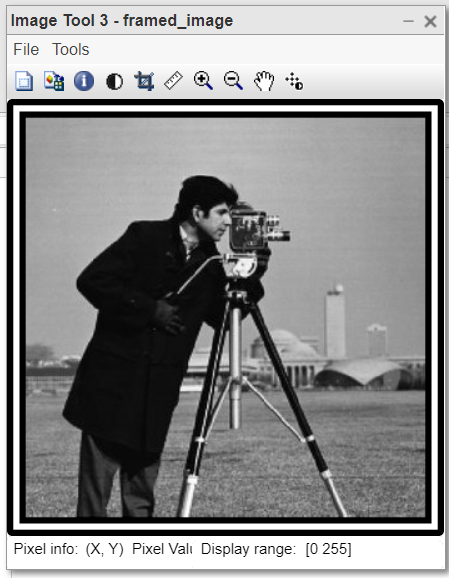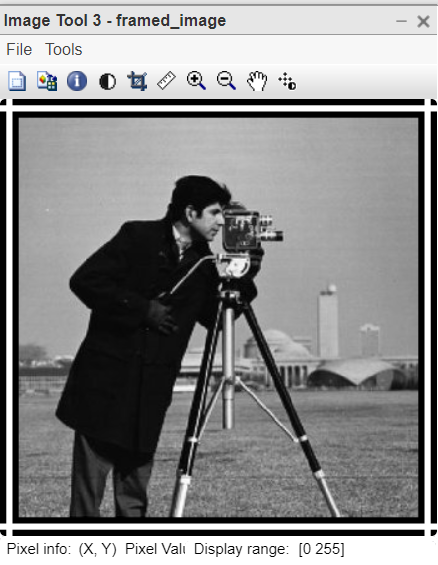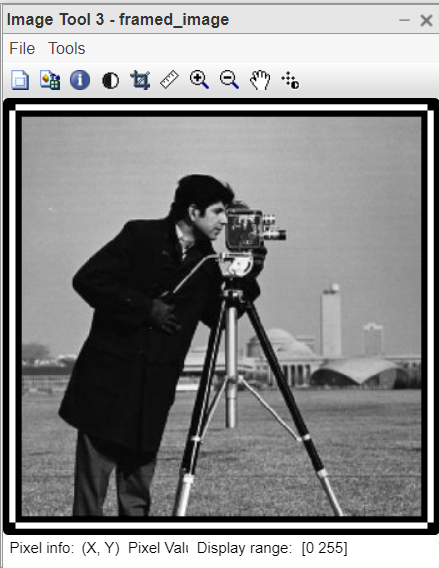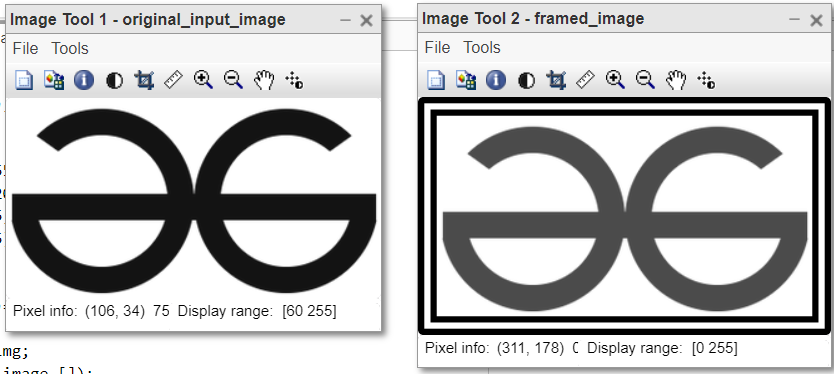Code Explanation:

• frame=zeros(x+30,y+30); This line creates the new image with black background.
• frame(6:10,11:y+20)=255; This line adds top-horizontal frame line.
• frame(x+21:x+25,11:y+20)=255; This line adds bottom-horizontal frame line.
• frame(6:x+25,6:10)=255; This line adds left-vertical frame line.
• frame(6:x+25,y+21:y+25)=255; This line adds right-vertical frame line.
• frame(16:x+15,16:y+15)=img(:,:); This line adds image in middle.
• imtool(framed_image,[]); This line displays the framed image.

Example:

## Matlab

 `% MATLAB CODE Add Coloured Frame to the RGB image.``function` `AddColouredFrame(img)`` ` `% This function takes colour image only.``[x,y,~]=size(img);``framed_img(x+20,y+20,3)=0;``framed_img=uint8(framed_img);``framed_img(11:x+10,11:y+10,:)=img(:,:,:);`` ` `% Horizontal Yellow lines on Top and bottom of 3 px.``framed_img(1:3,:,1:2)=255;``framed_img(x+18:x+20,:,1:2)=255;`` ` `% Vertical Yellow lines on Left and Right of 3 px.``framed_img(:,1:3,1:2)=255;``framed_img(:,y+18:y+20,1:2)=255;`` ` `% Horizontal Cyan lines on Top and bottom of 4 px.``framed_img(4:7,4:y+17,2:3)=255;``framed_img(x+14:x+17,4:y+17,2:3)=255;`` ` `% Vertical Cyan lines on Left and Right of 4 px.``framed_img(4:x+17,4:7,2:3)=255;``framed_img(4:x+17,y+14:y+17,2:3)=255;`` ` `% Horizontal Red lines on Top and bottom of 3 px.``framed_img(8:10,8:y+13,1)=200;``framed_img(x+11:x+13,8:y+13,1)=200;`` ` `% Vertical Red lines on Left and Right of 3 px.``framed_img(8:x+13,8:10,1)=200;``framed_img(8:x+13,y+11:y+13,1)=200;``imtool(framed_img,[]);``end`` ` `% Utility code%``k=imread(``"logo.png"``);``AddColouredFrame(k);`

Output: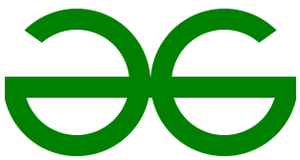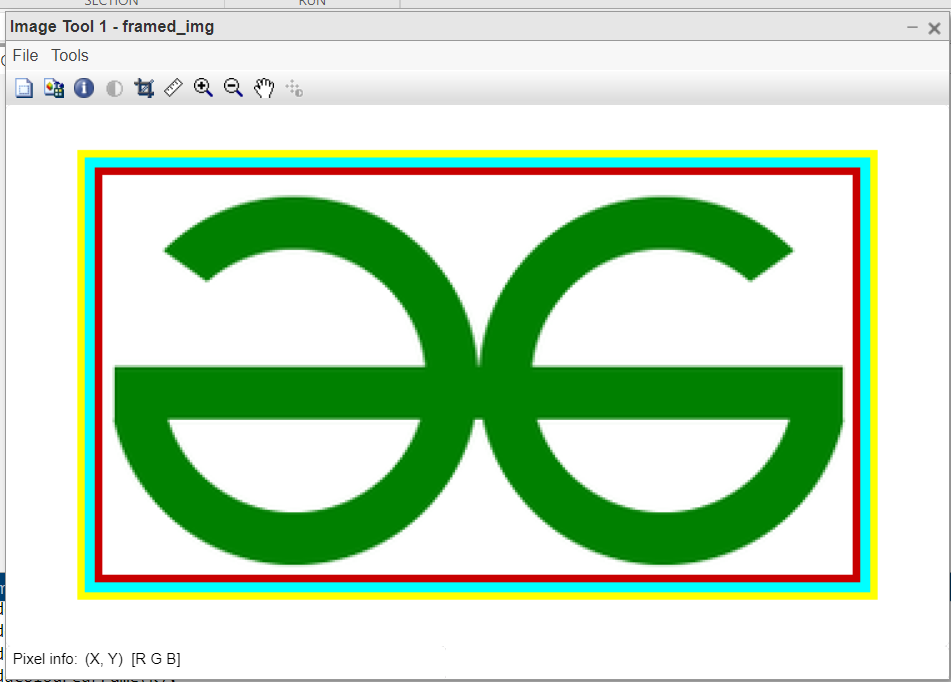Code Explanation:

• framed_img(x+20,y+20,3)=0; This line creates the empty image with black background.
• framed_img(11:x+10,11:y+10,:)=img(:,:,:); This line adds the input image into the middle of frame.
• framed_img(1:3,:,1:2)=255; This line adds 3 pixel thick Horizontal Yellow line at Top.
• framed_img(x+18:x+20,:,1:2)=255; This line adds 3 pixel thick Horizontal Yellow line at bottom.
• framed_img(:,1:3,1:2)=255; This line adds 3 pixel thick Vertical Yellow lines on Left.
• framed_img(:,y+18:y+20,1:2)=255; This line adds 3 pixel thick Vertical Yellow lines on Right.
• imtool(framed_img,[]); This displays the framed image.